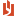## TS module 16 ARIMA(0,1,1) forecasting

Author
Message
NEASSupreme BeingPosts: 4.2K, Visits: 1.2K

TS module 16 ARIMA(0,1,1) forecasting

An ARIMA(0,1,1) process (= an IMA(1,1) process) is the cumulative sum of an MA(1) process. Final exam problems on ARIMA(0,1,1) processes can be solved by first principles.

For an MA(1) process, Cryer and Chan use è instead of è1. For consistency of notation with moving average processes of higher order (MA(2), MA(3), and so forth), the discussion forum postings often use è1.

Read the subsection of MA(1) processes in Cryer and Chan, chapter 9, pages 197-198; know equation 9.3.21 on page 197. See also the equation at the bottom on page 202: for the IMA(1,1) model, øj = 1 – è for j ≥ 1.

**Exercise 16.1: ARIMA(0,1,1) Process

A time series follows an ARIMA(0,1,1) process = IMA(1,1) process. The forecast and actual values for Periods 48, 49, and 50 are shown below. The forecasts are one period ahead forecasts: ŷ48(1) for Period 49 and ŷ49(1) for Period 50. They are the expected values for those periods.

 Period Forecast Values Actual Values 48 80.5 81.5 49 82.0 84.0 50 83.0 84.8

A.      What are the forecasts and values of the underlying MA(1) process (the first differences)?

B.      What are the residuals of the underlying MA(1) process (the first differences)?

C.      What are the forecasts for Periods 49 and 50 as linear combinations of ì and è1?

D.     What is the mean ì of the MA(1) process of first differences?

E.      What is the è1 of the MA(1) process of first differences?

F.      What is the forecast for Period 51?

Part A: The table below show the MA(1) forecasts and actual values.

The MA(1) actual values are the first differences of the ARIMA(0,1,1) values.

The MA(1) forecasts are the ARIMA forecasts minus the previous ARIMA actual value.

 Period Forecast Values Actual Values 48 80.5 – Y47 81.5 – Y47 49 0.5 2.5 50 -1.0 0.8

We don’t know the ARIMA(0,1,1) value for Period 47. We show the expression in the table above to compute the residual for Period 48 (see below).

Part B: The residual is the actual value minus the forecast value.

 Period Forecast Values Actual Values Residual 48 80.5 – Y47 81.5 – Y47 1.0 49 0.5 2.5 2.0 50 -1.0 0.8 1.8

The residual for the first differences equals the residual for the original time series. We can compute the same residuals from the ARIMA process.

 Period Forecast Actual Residual 48 80.5 81.5 1.0 49 82.0 84.0 2.0 50 83.0 84.8 1.8

Part C: Each forecast equals ìè1 × åt-1

For Period 49, the forecast of the first difference is 82.0 – 81.5 = 0.5. This forecast equals

the mean – è1 × the residual of Period 49

ìè1 × å48

0.5 = ìè1 × 1.0.

For Period 50, the forecast of the first difference is 83.0 – 84.0 = –1.0. This forecast equals

the mean – è1 × the residual of Period 49

ìè1 × å49

–1.0 = ìè1 × 2.0.

Parts D and E: We solve the simultaneous linear equations for ì = 2 and è1 = 1.5.

Part F: The residual in Period 50 is 84.8 – 83.0 = 1.8.  The first difference for Period 51 is 2.0 – 1.5 × 1.8 = -0.700 . The forecast for Period 51 is 84.8 – 0.70 = 84.100.

Exercise 16.2: ARIMA(0,1,1) Model

An ARIMA(0,1,1) model for a time series of 60 observations, yt, t = 1, 2, …, 60, has ì = 0 and θ1 = 0.4.  The forecast of the next observation, y61, is 38. The actual value of y61 is 39. We continue to use the same ARIMA model.

What is the new forecast of y62?

Solution 16.2: The residual in period 61 is 39 – 38 = 1. The forecasted first difference is 0 – 0.4 × 1 = – 0.4. The forecasted value for Period 62 is 39 + –0.4 = 38.6.

AttachmentsTS module 16 ARIMA(0,1,1) forecasting.pdf (1.7K views, 64.00 KB)
##### Merge Selected
Merge into selected topic...

Merge into merge target...

Merge into a specific topic ID...